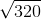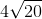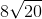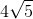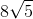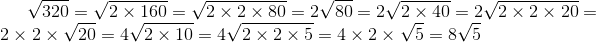# PSAT Math : How to find the common factors of squares

## Example Questions

### Example Question #1 : How To Find The Common Factor Of Square Roots

Solve for: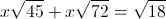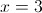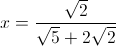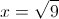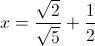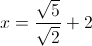Explanation:Notice how all of the quantities in square roots are divisible by 9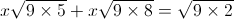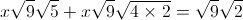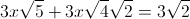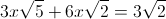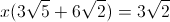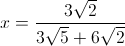Simplifying, this becomes### Example Question #1 : Factoring And Simplifying Square Roots

If m and n are postive integers and 4m = 2n, what is the value of m/n?

2

4

8

1/2

16

1/2

Explanation:
1. 2= 4. Also, following the rules of exponents, 4= 1.
2. One can therefore say that m = 1 and n = 2.
3. The question asks to solve for m/n. Since m = 1 and n = 2, m/n = 1/2.

### Example Question #2 : Factoring And Simplifying Square Roots